Learn data science and machine learning by building real-world projects on Jovian

Factors that contribute to National Happiness

The goal of this analysis is to determine the factors that correlate to national happiness. I retreived this data from Kaggle. I am going to download the data set. Retrieve it, clean it and see if I can find a correlation between various factors -like income for instance- and happiness.

import seaborn as sns #seaborn the python graphing module for more advanced graphing
import matplotlib #matplotlib the python graphing module for more basic graphing
import matplotlib.pyplot as plt #matplotlib the python graphing module for more basic graphing
import numpy as np #numpy the python module that provide you with math functions and functions for arrays
import statsmodels.api as sm #statsmodels.api the python module that contain functions allowing you to apply statistical models
%matplotlib inline

sns.set_style('darkgrid')
matplotlib.rcParams['font.size'] = 14
matplotlib.rcParams['figure.figsize'] = (12, 5)
matplotlib.rcParams['figure.facecolor'] = '#00000000' #short cuts to customize the look of plots for graphing
!pip install jovian opendatasets --upgrade --quiet
100%|██████████| 36.8k/36.8k [00:00<00:00, 25.7MB/s]

pwd
'/home/jovyan'
# select directory
data_dir = './world-happiness'
import os
os.listdir(data_dir) #files in the directory
['2018.csv', '2015.csv', '2017.csv', '2019.csv', '2016.csv']

Let us save and upload our work to Jovian before continuing.

project_name = "thanzin-zerotopandas-course-project-happiness-1" # my project name
import jovian
import pandas as pd
happy_raw_df

This dataset represents happiness rankings among countries

Question: How are countries ranked by happiness?

Methodology: A method called a Cantril ladder is being used here. The way it works is that it asks respondents to rate their own current lives on a scale of 10 to 0, with the best possible life being 10 and the worst possible life being 0. The countries are then ranked in descending order based on the average score.

Metrics: The columns after the happiness score are factors that estimate how much each variable contribute to the overall score for that particular country compared to a hypothetical country that has the lowest score in each of the 6 factors. In other words, it tells you how much do each of these six factors are positively impacting the the average happiness score in each country.

happy_raw_df.shape #we see that the dataset has 156 rows and 9 columns
(156, 9)
happy_raw_df.isnull().values.any() #check for any missing values in the dataframe; there are none and the dataset is complete
False
Note: I will rename 'Perceptions of corruption' as 'Absence of Corruption' since that is what it is referred to as in the kaggle description. Additionally, it can clear up confusion as a higher score actually means that the public perceive there to be less corruption and that is contributing to the happiness score; additionally I will rename the score as happiness to make it more clear
happy_raw_df.rename(columns= {'Perceptions of corruption': 'Absence of Corruption', 'Score':'Happiness'}, inplace= True)

happy_raw_df

Let us find the max and min of each column to see if there are any data value too high or low to make sense. For example, negative values or a metric score that is higher than the overall score

happy_raw_df.max(axis= 0)   #the highest value of each column
Overall rank                         156
Country or region               Zimbabwe
Happiness                          7.769
GDP per capita                     1.684
Social support                     1.624
Healthy life expectancy            1.141
Freedom to make life choices       0.631
Generosity                         0.566
Absence of Corruption              0.453
dtype: object
happy_raw_df.min(axis= 0)    #the lowest value of each column
Overall rank                              1
Country or region               Afghanistan
Happiness                             2.853
GDP per capita                          0.0
Social support                          0.0
Healthy life expectancy                 0.0
Freedom to make life choices            0.0
Generosity                              0.0
Absence of Corruption                   0.0
dtype: object
Note: for the min, the zeros mean that in each column, there is at lease one country where a certain metric contributes nothing to the overall happiness score of a country
happy_raw_df.describe() #the descriptive statistics of the dataset
#filter out of indices(rows) that feature a zero value in each column
zero_metric = happy_raw_df[(happy_raw_df['GDP per capita'] == 0)|
(happy_raw_df['Social support'] == 0)|
(happy_raw_df['Healthy life expectancy'] == 0)|
(happy_raw_df['Freedom to make life choices'] == 0)|
(happy_raw_df['Generosity'] == 0)|
(happy_raw_df['Absence of Corruption'] == 0)]
zero_metric

It's very unlikely that any metric contributes nothing to the overall score. Zero values can be explained by standard deviation since the actual numbers can be a little higher or lower. For this reason, I will turn all 0.00 values to NaN to make the dataset slighty more accurate.

happy_df = happy_raw_df.replace(to_replace= 0, value= np.nan) #replace all zeros in the dataframe with NaN
happy_df.describe()

Question 1: Many people believe that the GDP per capita of a country is the main contributing factor to overall well being. How true is this?

sns.scatterplot(x=happy_df['GDP per capita'] ,y= happy_df['Happiness']); #scatter plot of gdp per capita against happiness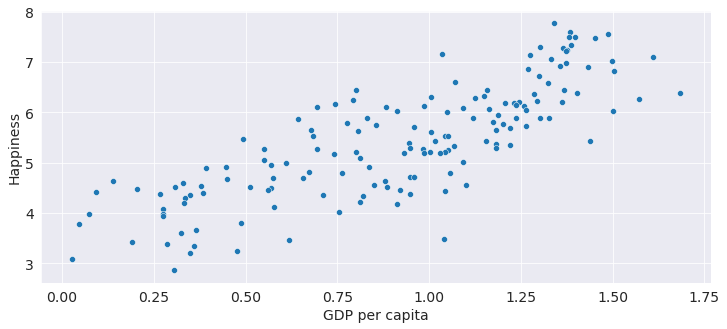sns.regplot(x=happy_df['GDP per capita'] ,y= happy_df['Happiness']); #the same plot but with the best fit line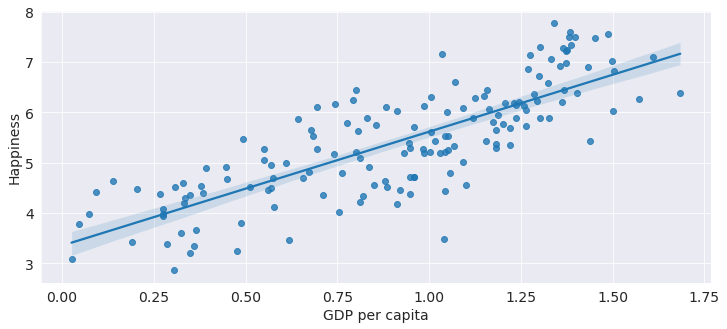However before we apply the correlation coefficient we must first make sure that the data nomially distributed if I want to use Pearson's coefficient

sm.qqplot(np.random.normal(0,1, 1000), line ='s'); #this is a plot of a quantile v quantile plot which is a plot that measures
#if a series a normally distributed
# This is what it should look like if the array is normally distibuted with most of the points on the line

#line = 's' means that the standardized line is applied
/opt/conda/lib/python3.9/site-packages/statsmodels/graphics/gofplots.py:993: UserWarning: marker is redundantly defined by the 'marker' keyword argument and the fmt string "bo" (-> marker='o'). The keyword argument will take precedence. ax.plot(x, y, fmt, **plot_style)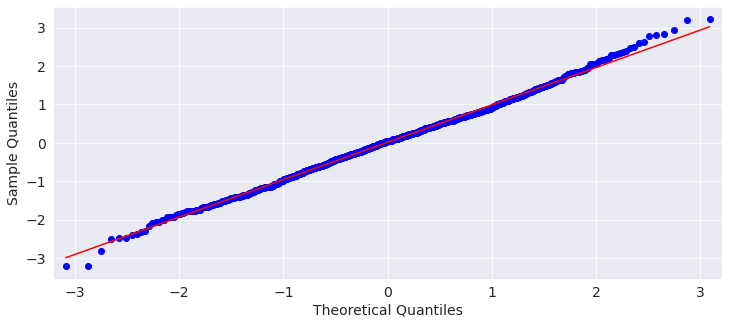The quantiles of the input array is plotted against the quantile of a theoretical normal distribution to see whether the input array is normally distributed

sm.qqplot(happy_df['GDP per capita'], line ='45'); #qq plot of our gdp per capita array, which is not a normal distribution

#I cannot use line = 's' in this case because the points are too far from a normal distribution and the line will not appear.
#However, I placed the 45 degree line there to demonstrate where the blue dots should be if the series was normally distributed
/opt/conda/lib/python3.9/site-packages/statsmodels/graphics/gofplots.py:993: UserWarning: marker is redundantly defined by the 'marker' keyword argument and the fmt string "bo" (-> marker='o'). The keyword argument will take precedence. ax.plot(x, y, fmt, **plot_style)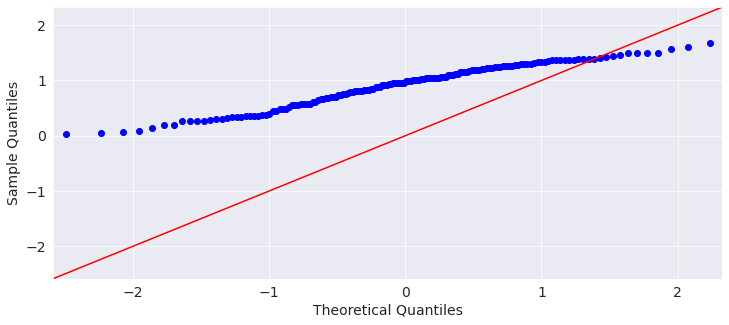sm.qqplot(happy_df['Happiness'], line ='s'); #happiness metric is a normal distribution
/opt/conda/lib/python3.9/site-packages/statsmodels/graphics/gofplots.py:993: UserWarning: marker is redundantly defined by the 'marker' keyword argument and the fmt string "bo" (-> marker='o'). The keyword argument will take precedence. ax.plot(x, y, fmt, **plot_style)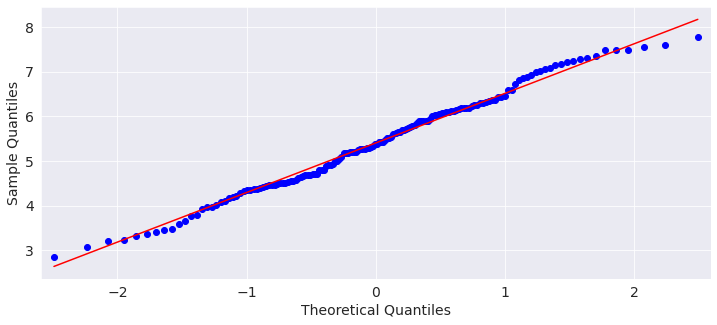Since both variables have to be normally distributed. Using Pearson's correlation coefficient is not appropriate. Instead I should use Spearman's correlation coefficient since that does not require both variables to be normally distributed.

corr_spearman = happy_df.corr(method = 'spearman') #apply spearman's correlation coefficient to the dataframe
corr_spearman

Question 2: Which metric overall contribute the most to the happiness score of a nation on average?

happy_df
happy_df.describe() #descriptive stats

It seems that on average(mean) social support contribute most to well being. Let us take a look at it in more detail

sns.scatterplot(x=happy_df['Social support'] ,y= happy_df['Happiness']); #scatter plot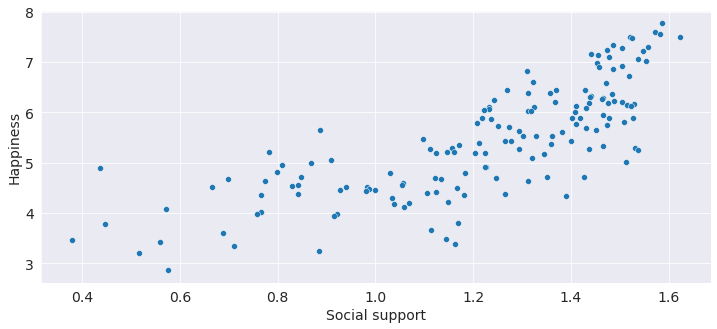sns.regplot(x=happy_df['Social support'] ,y= happy_df['Happiness']); #best fit line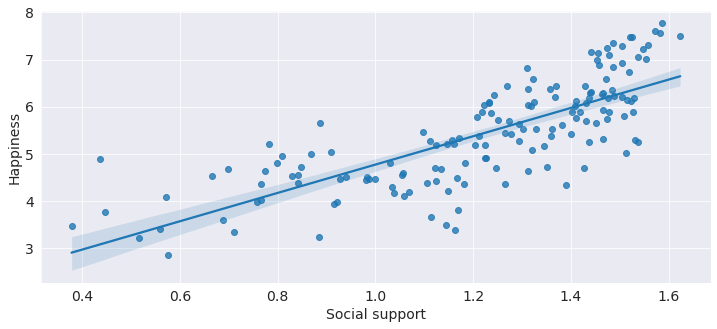corr_spearman

Even though social support contributes most of well being, it has the same correlation or lower compared to the gdp per capita. The reason this maybe the case is because social support is more consistantly higher.

happy_df.describe()

Question 3: Specifically how does the United States fare compared to the world average?

us_filt = happy_df[happy_raw_df['Country or region'] == 'United States'] #filter out all countries other than the US
#create new dataframe
us_filt = us_filt.iloc[:,[2,3,4,5,6,7,8]]    #Select for the metrics in the dataframe
us_filt['Region'] = 'United States'          #Create a new column named 'region' and add the US to it
cols = us_filt.columns.tolist() #turn the column series to a list
cols = cols[-1:] + cols[:-1]    #so that you can use indexing to put 'region' column in first place and move other columns up

us_filt = us_filt[cols] #apply indexing to dataframe
us_filt #data frame for US only
plt.xticks(rotation=90) #90 degree ticks
plt.title('United States') #title
plt.xlabel('Metrics') #xlabel
plt.ylabel('Metric Values') #ylabel
sns.barplot(data = us_filt); #barplot of the dataset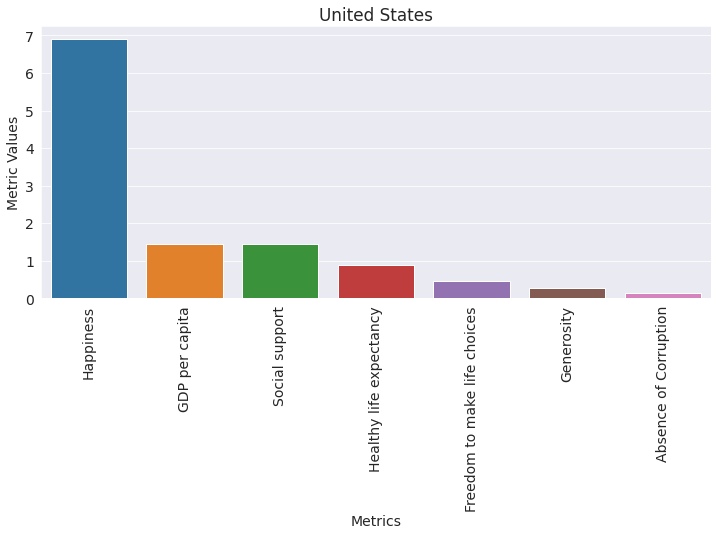This is a barplot showing the distribution of metrics for the US. It seems that GDP per capita, social Support, and life expectancy matter most to well being. While absence of corruption does very little. How does this compare to other nations?

#calculate the mean of all columns assign each value to a variable
global_score = happy_df['Happiness'].mean(axis = 0)
global_gdp = happy_df['GDP per capita'].mean(axis= 0)
global_social = happy_df['Social support'].mean(axis= 0)
global_health = happy_df['Healthy life expectancy'].mean(axis= 0)
global_freedom = happy_df['Freedom to make life choices'].mean(axis= 0)
global_generosity =  happy_df['Generosity'].mean(axis= 0)
global_corruption = happy_df['Absence of Corruption'].mean(axis= 0)

#and create a dictionary with keys and values
data = {'Happiness':[global_score],
'GDP per capita': [global_gdp],
'Social support': [global_social],
'Healthy life expectancy': [global_health],
'Freedom to make life choices': [global_freedom],
'Generosity': [global_generosity],
'Absence of Corruption': [global_corruption]}

#a new dataframe of the created dictionary
global_filt = pd.DataFrame(data=data)
global_filt.insert(0,'Region','Global')
#insert the column 'region' with string 'global' to indicate the average of all countries for this dataframe
global_filt

us_world_compare_df = pd.concat([global_filt,us_filt]) #combine the global and us dataframes together to create a new dataframe

us_world_compare_df
#I cannot utilize current short form of the combined dataframe shown below
#I must first convert the short-form dataframe to a long-form dataframe so I can create a barplot for it
us_world_compare_longform_df = pd.melt(frame = us_world_compare_df, id_vars= 'Region', var_name= 'Metrics', value_name= "Values" )
us_world_compare_longform_df
plt.xticks(rotation=90) #90 degree ticks
sns.barplot(data = us_world_compare_longform_df, x= 'Metrics', y= 'Values', hue = 'Region');
#barplot comparing the metrics of the US versus the global average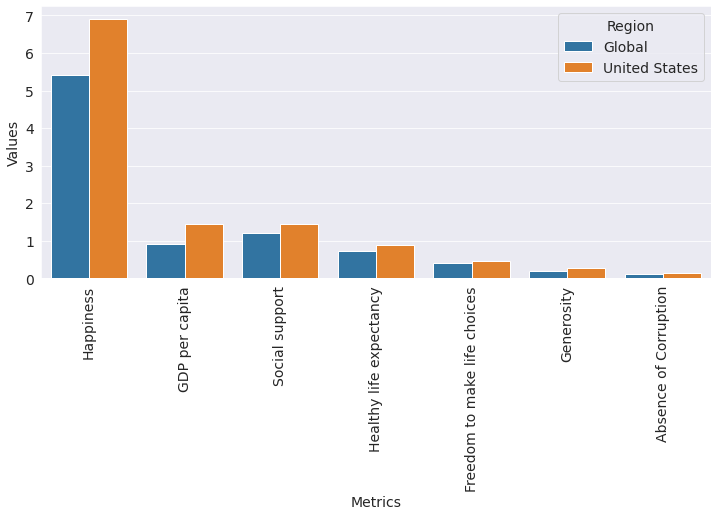Question 4: Is gdp per capita correlated with life expectency?

sns.scatterplot(x=happy_df['GDP per capita'] ,y= happy_df['Healthy life expectancy']); #scatterplot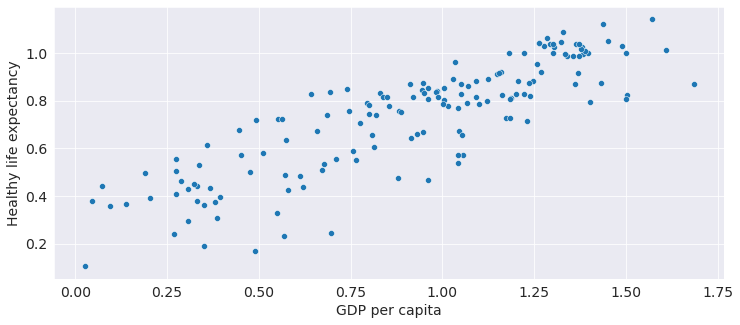sns.regplot(x=happy_df['GDP per capita'] ,y= happy_df['Healthy life expectancy']); #the line of best fit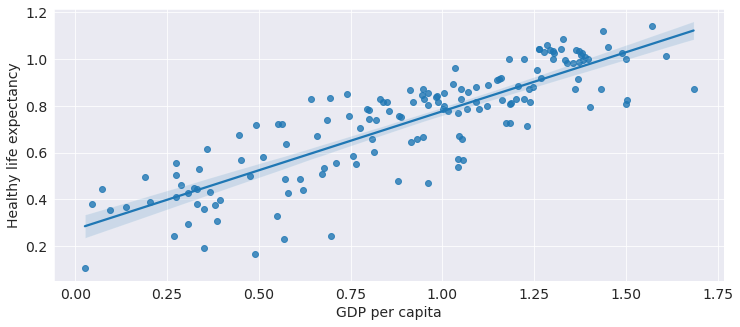corr_spearman

Question 5: What other relationships can we predict from the data?

sns.heatmap(corr_spearman) #create a heatmap based on spearman's correlation coefficients
#this shows the complete map of all correlation coefficients for every combination of all variables
plt.show()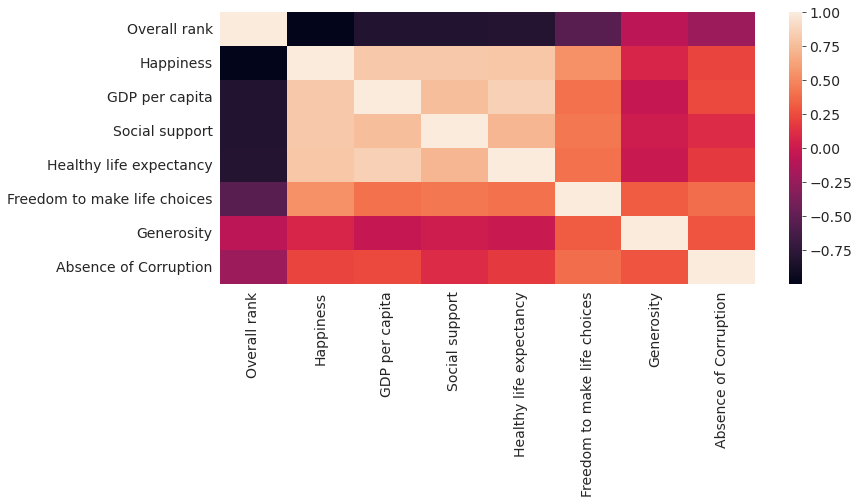Inferences and Conclusion

Future ideas:

-Finally, I want to make the same analysis for different years to see which nations' well beings increased or decreased

jovian.submit(assignment = 'thanzin-zerotopandas-course-project-2')